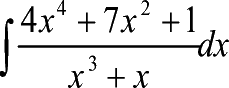Games
Problems
Go Pro!

Problems > Mr. T's Calculus > 2003

## Moldy Growth

A mold grows at a rate proportional to the amount present. Initially, its weight is 3 grams. After 2 days, its weight is 5 grams. How much does it weigh after 12 days?

## The oil tank problem

A fuel-oil tank is 10 feet long and has flat ends that are perpendicular to the ground surface. Cross-sections parallel to the flat ends have the shape of the ellipse x2/9 + y2/36 = 1. If the fuel oil in the tank is 9 feet deep, what is the volume of the fuel oil in the tank?

Show the integrals you use to solve this problem. You MAY do a numerical integration, rather than applying the Fundamental Theorem.

## Find the antiderivative

Find the antiderivative shown WITHOUT the use of a calculator or computer system that does algebra. Explain your method clearly.Like us on Facebook to get updates about new resources